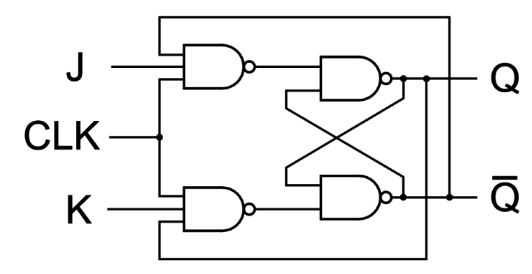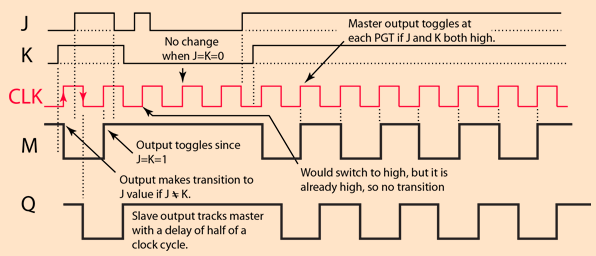# J K Flip Flop Explained in Detail

Flip – flops are one of the most fundamental electronic components. These are used as one-bit storage elements, clock dividers and also we can make counters, shift registers and storing registers by connecting the flip flops in particular sequences. These flip flops use feedback concept to create sequential logic where the previous state affect future states (unlike combinational circuit).

An essential characteristic of flip flops is that it changes its state whenever there is a positive or negative transition on the control signal. Also, we need to define the input-output relationship here. The common types of flip flops are as follows:

Among the above four, only D and J-K flip flops are available in the integrated IC form and have immense applications.

## Introduction

It is simply the most used flip flop among all the basic flip flops that we have. These J & K here are the two inputs and have been kept on the name of the inventor Jack Kilby. This is done in order to differentiate the design from other flip flops.

Now, we must get a question here.

Why do we need J K Flip flop?

The answer is in the shortcomings of the S R flip flop, which are –

• The undefined state of S R flip flop when both inputs are high (1).
• If set (S) or reset (R) changes the state while the enable (EN) input is high, then it might be possible that correct latching action may not happen.

## The basic J K Flip Flop

A gated S R flip flop with the addition of a clock input circuitry is basically the J k flip flop. This circuit prevents the invalid output condition which occurs when both inputs are high. The new addition here gives us four possible outputs of the flip flop. The output may be – No Change, Logic 0, Logic 1 & Toggle.JK Flip Flop

In the above circuit, we can see that the output is fed back to the enabling NAND gates. This makes the toggling action possible at J=K=1. But here we have a problem to deal with, its with timing. The timing pulse must be for very less time because if Q changes before the clock pulse goes off then it can drive the circuit into an oscillation condition called ‘Racing’.

As we know in the modern technology, the ICs speed is quite high, so this simple J K flop flop can’t be used in practical. Now to overcome this, we need an extra circuitry with four more NAND gates to create a Master Slave J K Flip Flop. It has two gated S R flip flops as a latch which suppresses the oscillation, i.e., racing.

### Truth Table for J K Flip Flop

 Clk J K Qn+1 X X X No change 1 0 0 No change 1 0 1 0 1 1 0 1 1 1 1 Toggle (Q’)

Here, ‘X’ is the don’t care condition. Well the last row of the above table solves the problem of invalid output of SR flip flop. But as discussed above a new problem arises, i.e., racing. Let us now try to understand that what exactly we mean by the term ‘Racing’.

## Racing in JK Flip Flop

At J=K=1, we achieved toggling by proving a feedback of output to the enabling NAND gates. It is one of the desired behavior but in general we would like the number of toggles to be controlled by the clock pulses as enablers. Therefore, we can predict and control the output.Racing condition

For this simple J K flip flop, the toggling will occur as soon as J=k=1 and clock is high (1) and the rate of toggling would be determined by the propagation delay around the circuit. Thus, this makes the output of the flip flop unpredictable at anytime from the clock state. This is called ‘race around condition’ or racing.

Therefore, to avoid such a condition we use a Master Slave circuit arrangement. Using this makes the transmission of the J value delayed by half a clock cycle to the output and thus not immediately fed back to the input side.

### Master – Slave JK Flip Flop

The clock’s positive going transition enables the switching of the output Q. But this enable condition, doesn’t persist for an positive phase of the clock. Only the inputs J & K can’t cause a transition but their values at the time of positive going transition (PGT), determines the output as per the truth table. This circuit can be counted as an application of the J K flip flop.Master Slave JK Flip Flop

Clearly, the Master Slave J K flip flop was developed to give a more stable circuit with the same function as the basic J K flip flop and no racing condition. This modified circuit has two gated SR flip flops used as latches in a way so that it suppresses the racing around or racing behavior. Also, we can see it other way round like two J K flip flops ties together with the second driven by an inverted clock signal.

As the clock makes a positive transition, the master section gets triggered. But not the slave circuit as its clock is inverted. On the downward transition (at a half cycle of the clock, the inverted clock has a positive transition and triggers the slave section.Then Q, (i.e., the final output) tracks the output of the master section after a half cycle of the clock.Let us now learn  some basic differences between latches and Flip Flop.

## Latch V/s Flip Flop

The difference between a flip flop and a latch is that flip flop is edge triggered (changes state only when a control signal goes from high to low or low to high). While, on the other hand latches are level triggered (output changes as soon as input changes). Let us list out few differences between the two as follows:

 Flip Flop Latch It is a pair of latches (slave and master flops). The signal propagates only on rising or falling edge called as hard barrier. It is sensitive to pulse transition. It is like buffer because output is directly connected to input when enable is high. Therefore, latch is sensitive to pulse duration (soft barrier). More gates as flip flop contains two latches. Less number of gates are used. More power consumption as more gates. Less power consumption. As more gates, therefore, it is slow. Speed is better. Easy to check design timing using STA (Static Timing Analysis) tools. More hand calculations and tool manipulation is required for assurance that they meet timing. Data launched on one rising edge, must setup before next rising edge. System might fail, if it arrives late. On early arrival, time gets waste due to hard edges in flops. As long as each loop completes in one cycle, cycle – borrowing to gain more setup time on the next register stage. Designers consider latches to adjust timing mismatch, to meet the timing in the design. Highly accurate wire load  model and process are required for flip flops. As latches are less sensitive, therefore, it reduces the impact of inaccuracy of wire load models and process variation. Flip flops that can be scanned (controllable and observable) should be used in DFT. To avoid unpredictable behavior in DFT, latches need a lockup state at the clock domain crossings in the scan chain. A flip flop is edge triggered and changes state only when a control signal goes from low to high or high to low. On the other hand, latch is an asynchronous block. Therefore, we must ensure race free combinational functions, which generate input signals for the latch. Else glitches may be generated causing hazards to the system. Designs made up of flip flops are robust. Conversely, designs based on latch are noisy as any noise in the enable signal disrupts the latch output easily.

### Applications of Flip Flops

There are various applications of the flip flops as they are one of the most basic unit in electronic circuits. Some are specified as follows:

• Shift Registers
• Frequency dividers
• Storage registers
• Counters
•
•
•
•
•
•